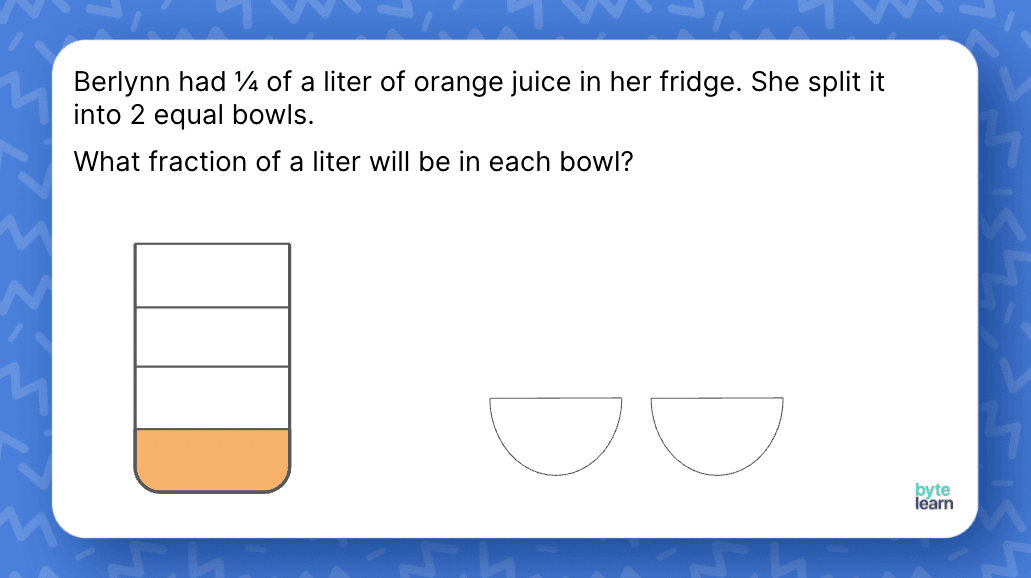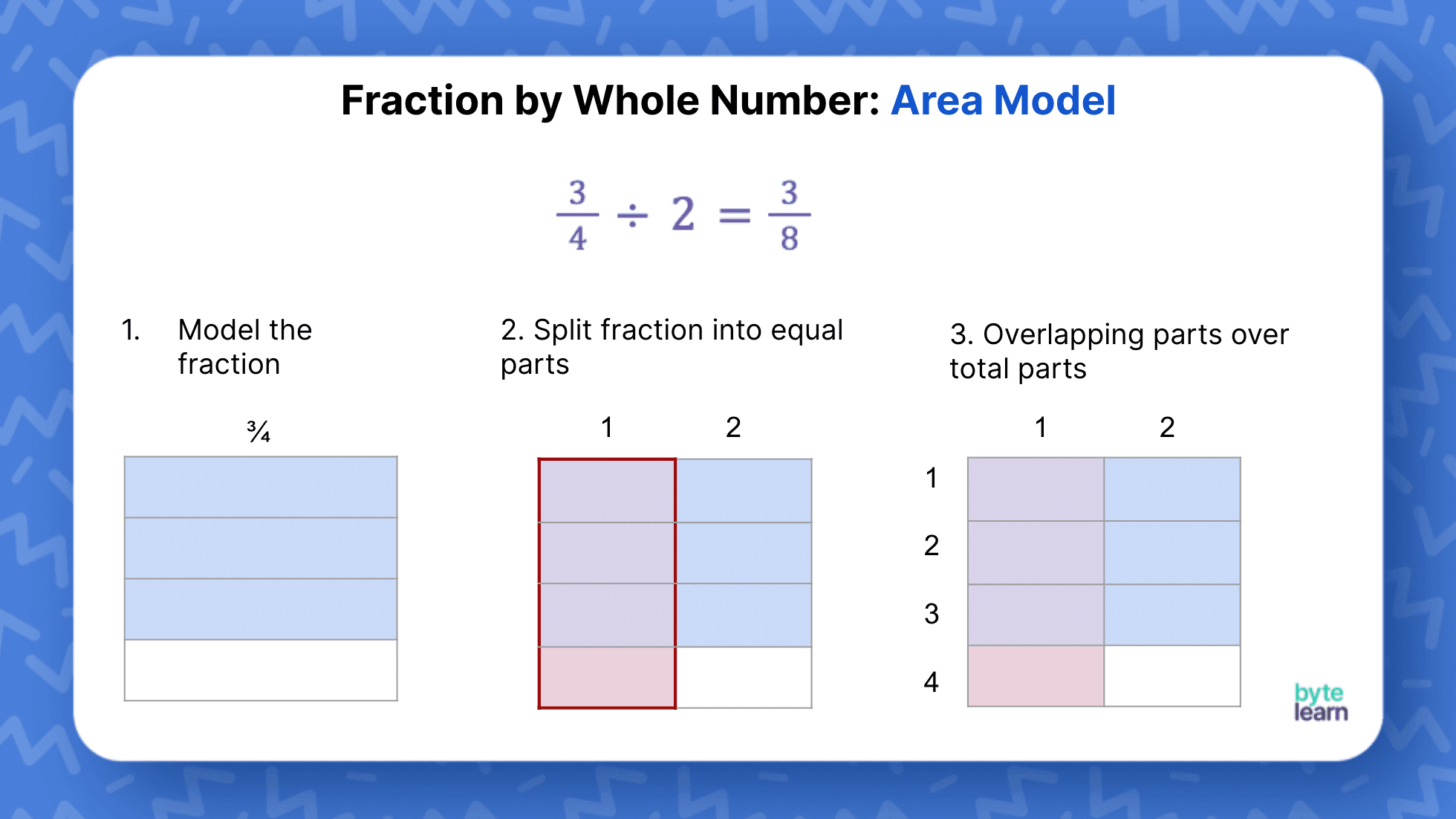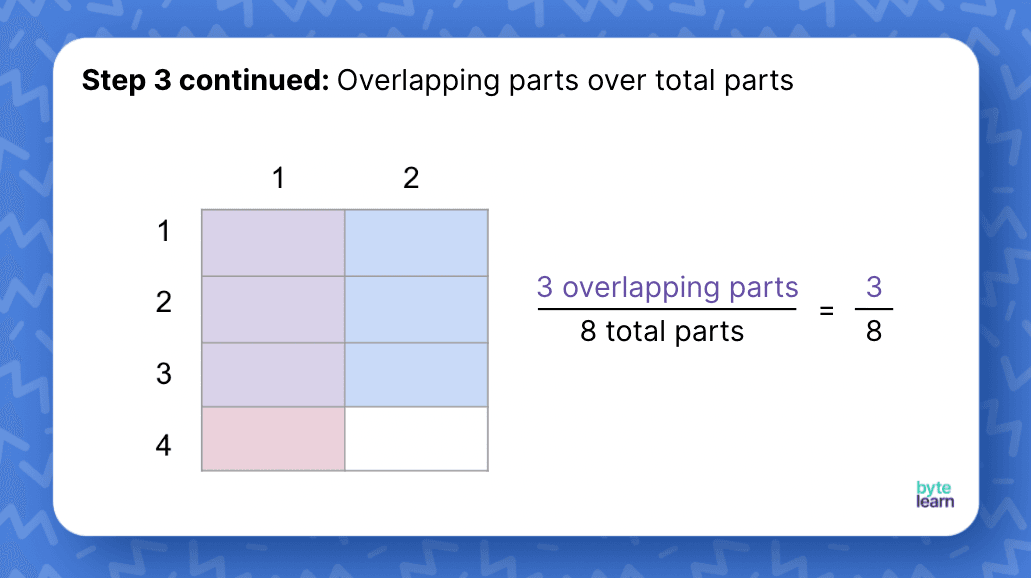ResourcesTestimonials
Plans
ResourcesTestimonials
PlansLesson plan

# Dividing Fractions With Models Lesson Plan - 6th Grade Math

## Overview

In this lesson, we’ll introduce the concept of dividing fractions for 6th graders using area models and number lines. Students will use area models for dividing a fraction by a whole number and number lines for dividing a whole number by a fraction. You can expect this lesson to take one 45-minute class period.

Number System
6.NS.A.1

## Objective

Students will be able to divide a whole by a fraction and a fraction by a whole using models.

## Materials

• Bell Ringer
• Slideshow
• Matching Activity
• Online Practice

## How to Teach Dividing Fractions Using Models

### Overall flow

You will start the lesson by giving a problem that focuses on quotative division - where we know the size of each group and the total, and we want to find the number of groups. You will build on student thinking by introducing division using a number line.

You will then move on to a problem about partitive division - where we know the number of groups and the total and want to find the size of each group.  Here you will introduce the area model to think about the size of each group.

### Dividing a whole number by a fraction

Start students off by giving them the word problem on the first slide. Allow them time to work independently or with a partner to find the answer.There are many ways students might find the answer, so you’ll want to have students explain their reasoning to the class. Different methods might include:

• Drawing the cookies and splitting each one into fourths, then counting the smaller pieces.
• Multiplying 6 by 4, knowing that each cookie can feed 4 students and there are 6 cookies in all.
• Writing out 6 \div \frac{1}{4} and solving.

It’s important to point out to students that they could have used different methods, but they landed at the same answer, 24 students!

### Is this a multiplication problem or a division problem?

You want to set up the ground for linking division to multiplication. Show slide 2 for a conversation about if this is a multiplication problem or a division problem. Students may recognize that we can solve this problem using multiplication (6 \times 4) since there are 4 one-fourths in each whole. Some students might write this as \frac{1}{4} \times 24 = 6. Some students might say that we are dividing 6 cookies into fourths, so this is a division problem. Acknowledge that we can this problem in so many different ways.

### Translate to a number line

Build on their work on the cookie problem by translating it to a number line. Ask them what relationship do they see between the number line and the cookie problem.

You will want to explicitly talk about “You can think of 6 \div \frac{1}{4} as how many times does \frac{1}{4} fit into 6.” Using this language consistently with students helps them to see division not just as an operation.

### More difficult problems on the number line

Transition to the next example, where we’ll use a number line to work through 3 \div \frac{3}{4}.

We start with a number line from 0 to 3. We want to ask the question, “how many jumps of \frac{3}{4} does it take to get to 3?” Ask a student to come to the board to draw the jumps. Students might be tempted to jump by \frac{1}{4}. Whatever jumps the student makes, you want to ask the other students, “Do you agree?”

It is quite likely some students will point out that the number of jumps tells us our answer!  If not, pose the question, “What does the number of jumps tell us about 3 divided by \frac{3}{4}?”

### Dividing a fraction by a whole number

Now it’s time for another real-world example. Display slide 5. Just like the cookie example, we don’t want to give students any additional context, just allow them to work through and use logic to solve. You can have them work independently at first, then check their answer with a partner.

When students are done, you’ll want to go over different methods they might have used. Here are some different ways students might have thought about this:

• Students might have shown the orange juice container split into fourths, then split that into 2 parts.
• Thinking about the question as half of \frac{1}{4}, and knowing that would be \frac{1}{8}.
• Thinking about the question as \frac{1}{4} divided by 2, and knowing that would be \frac{1}{8}.

### Introduce the area model

After you go through different methods, use the next few slides to give an informal introduction to the area model. If a student has already used the area model to explain their thinking, use their work - ask the other students to analyze what this student has done.Explain that this orange juice container is split into fourths because we know that Berlynn is using \frac{1}{4} of the liter of orange juice. Now, we’ll want to split that into 2, as shown in this next slide:

This makes it easier for students to recognize that \frac{1}{8} of the orange juice will go in one bowl, and \frac{1}{8} of the orange juice will go in the other bowl.

### Area model example

Transition to the next example, where we’ll use an area model to divide \frac{3}{4} by 2.Ask students what they notice about how the area model represents \frac{3}{4} \div 2. Students are likely to make a connection to the orange juice problem.

Here is what you want to talk about when describing the area model.

1. What does the whole square tell us? (the entire square represents the whole)
2. Why do we focus only on one column? (When we divide by 2, we are asking “how much is in one out of the two groups?”)
3. What do the overlapping parts tell us? (That 3 out of the 8 parts in a whole are in 1 group when we split \frac{3}{4} into two groups)### Matching activity

For this activity, students will work in pairs. Each student will take a turn in matching an equation to the number line or area model. Once all 6 matches have been found, check that the matches are correct before students solve the problems. Go over the answers with the class so that every student can see and make sense of the matches and answers. Here is a link to the materials needed for the activity.

## Dividing Fractions With Models Practice

After you’ve completed the examples with the whole class, it’s time for some independent practice! ByteLearn gives you access to tons of dividing fraction activities. Check out the online practice and assign to your students for classwork and/or homework!Dividing Fractions With Models Practice
Problem 1 of 4
<p>Follow the directions below to divide using an area model.</p><p>4/5 ÷ 3</p><selectivedisplay data-props='{"show_in_create":true, "show_in_problem_qa": true}'><p style="color:#808080"><i> Interactive: Students follow steps to create an exploratory area model.</i> </p></selectivedisplay>

View this practice